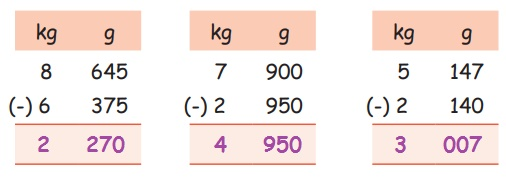Home | | Maths 4th Std | Exercise 4.2 (Estimate the weight of an object)

# Exercise 4.2 (Estimate the weight of an object)

Text Book Back Exercises Questions with Answers, Solution : 4th Maths : Term 2 Unit 4 : Measurements : Exercise 4.2 ; Estimate the weight of an object and verify using a balance

Exercise 4.2

1. Mohana bought 2kg 600g grapes and 1kg 450g guavas. How much more did Mohana buy grapes than guavas, by weight?

1) 150g   2) 1kg 150g 3) 1kg 200g 4) 4kg

2. Put >, < or = in the boxes provided below.

50g < 340g

640g < 800g

34kg > 22kg

1000g = 1kg4. Subtract:Activity

5. The weights of three babies are respectively 3kg 650g, 5kg 420g and 4kg 750g. Find their total weight?

Weight of first baby     = 3 kg 650 g

Weight of second baby  = 5 kg 420 g

Weight of third baby     = 4 kg 750 g +

Total weight                   = 13 kg 820 g

Answer: Total weight = 13kg 820g

6. A shopkeeper had 275kg 450g of coffee powder. He sold 80kg 475g. How much coffee powder left?

Total weight of coffee powder = 275 kg 450 g

Weight of sold coffee powder = 80 kg 475 g (-)

Weight of coffee powder left = 194 kg 975 g

Answer: The weight of the vegetables = 194kg 975g

Tags : Measurements | Term 2 Chapter 4 | 4th Maths , 4th Maths : Term 2 Unit 4 : Measurements
Study Material, Lecturing Notes, Assignment, Reference, Wiki description explanation, brief detail
4th Maths : Term 2 Unit 4 : Measurements : Exercise 4.2 (Estimate the weight of an object) | Measurements | Term 2 Chapter 4 | 4th Maths